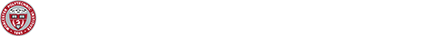# Biological and ecological models under stochastic perturbation, past dependence and spatial inhomogeneity: Modeling and longtime characterization

## Biological and ecological models under stochastic perturbation, past dependence and spatial inhomogeneity: Modeling and longtime characterization

• SpeakerNhu N. Nguyen from University of Connecticut
• Time: 04/22/21, 2-2:50pm
• Zoom : 992 7853 8762
• Abstract: The dynamics of many models in Biology and Ecology such as: epidemic models, tumor-immune models, chemostat models, prey-predator models, competitive models, and among others can be mathematically described. The earliest and simplest mathematical models are given by ordinary differential equations (ODE). Long-standing and important questions in mathematical biology are that: How is the long-time behavior of the system? Does one group of populations come extinct or persistent? Under which condition, the disease will be controlled in the epidemic systems?. In longtime, which species dominates the others? and among others. This talk focuses on modeling these above biological and ecological systems and answering such problems when the random factors (leading to stochastic system), past-dependence (leading to delay system), spatial inhomogeneity (leading to reaction-diffusion models) are taken into consideration, which are described under stochastic differential equations (SDEs), stochastic functional differential equations (SFDEs) and stochastic partial differential equations (SPDEs) framework. .